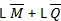# The trigonometric Fourier series for the waveform f(t) shown below contains

1.  Only cosine terms and zero value for the dc component

2.  Only cosine terms and a positive value for the dc component

3.  Only cosine terms and a negative value for the dc component

4.  Only sine terms and a negative value for the dc component

4

Only cosine terms and a negative value for the dc component

Explanation :
No Explanation available for this question

# If F9s)=L[f(t)]=2(s+1)/s2+4s+7 then the intial and final values of f(t) are respectively

1.  0, 2

2.  2, 0

3.  0, 2/7

4.  2/7, 0

4

2, 0

Explanation :
No Explanation available for this question

# A system is described by the differential equation d2y/dt2+5(dy/dt)+6y(t)=x(t)Let X9t) be a rectangular pulse given by X(t)={1 0

1.   e-2s/s(s+2)(s+3)

2.  1- e-2s/s(s+2)(s+3)

3.  e-2s/(s+2)(s+3)

4.  1-e-2s/(s+2)(s+3)

4

1- e-2s/s(s+2)(s+3)

Explanation :
No Explanation available for this question

# For an N-point FFT algorithm with N=2m, which one of the following statements is TRUE

1.   It is not possible to construct a signal flow graph with both input and output in normal order

2.  The number of butterflies in the mth stage is N/m

3.   In-place computation requires storage of only 2N node date

4.   Computation of a butterfly requires only one complex multiplication

4

It is not possible to construct a signal flow graph with both input and output in normal order

Explanation :
No Explanation available for this question

# The first six points of the 8-point DFT of a real valued sequence are 5, 1-j3, 0, 3-j4, 0 and 3+j4. The last two points of the DFT are respectively

1.   0, 1-j3

2.  0, 1+j3

3.  1+j3, 5

4.  1-j3, 5

4

0, 1+j3

Explanation :
No Explanation available for this question

# Consider the circuit given below with initial state Q0 = 1, Q1, Q2 = 0. The state of the circuit is given by the value 4 Q2 +2Q1 + Q0.­The correct state sequence of the circuit is

1.  1, 3, 4, 6, 7, 5, 2

2.  1, 2, 5, 3, 7, 6, 4

3.  1, 2, 7, 3, 5, 6, 4

4.  1, 6, 5, 7, 2, 3, 4

4

1, 2, 5, 3, 7, 6, 4

Explanation :
No Explanation available for this question

# The circuit shown in the figure given below

1.  Is an oscillating circuit and its output is a square wave

2.  Is one whose output remains stable i8n ‘1’ state

3.   is one having output semains stable ‘0’ state delay

4.  having a single pulse of 3 times propagation delay

4

Is an oscillating circuit and its output is a square wave

Explanation :
No Explanation available for this question

# A latch is to be defined inputs L and M (an L M latch). The table specifying the desired next state at a clock pulse is given in below. L M Q Q+ 0 0 0 0 0 0 1 0 0 1 0 0 0 1 1 0 1 0 0 1 1 0 1 1 1 1 0 1 1 1 1 0 The expression for the next state Q+ is

1.

2.

3.

4.

4Explanation :
No Explanation available for this question

# The DFT of vector [a, b, c, d] is the vector [α β γ δ]. Consider the product [p q r s]=[a b c d] The DFT of the vector [p q r s] is a scaled version of

1.   [α2 β2 γ2 δ2]

2.  √α √β √γ √δ

3.  [α+β β+δ δ+γ γ+α]

4.  [δ β γ δ]

4

2 β2 γ2 δ2]

Explanation :
No Explanation available for this question

# The region of convergence of the z-transform of a unit step function is

1.   |z|>1

2.  |z|

3.  (Real part of z)>0

4.   (Real part of z)

4